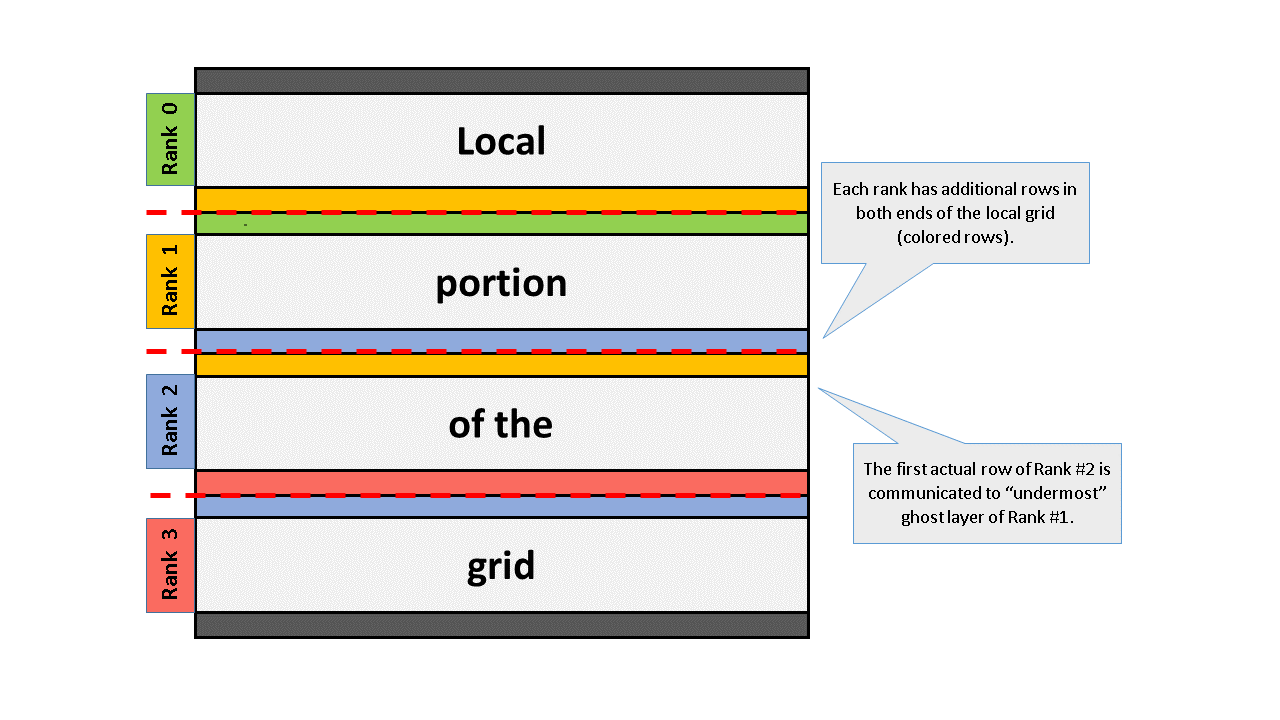4.23

# Bonus hands-on: Parallel heat equation solver

If you would like to further practice your parallel programming skills, we have as a final parallel programming hands-on exercise parallelization of the heat equation solver with MPI.

Source code for this exercise is located in mpi/heat-equation/

Parallelise the serial heat equation solver in numpy/heat-equation/ with MPI, by dividing the grid into blocks of rows and assigning one row block to one task. A domain decomposition, that is.

Note that the tasks are able to update the grid independently everywhere else than on the boundary rows – there the communication of a single row with the nearest neighbor is needed. This can be achieved by having additional ghost layers that contain the boundary data of the neighboring tasks. As the system is aperiodic, the outermost ranks communicate with a single neighbor, and the inner ranks with two neighbors.

Insert suitable MPI routines into the skeleton code in skeleton.py (search for “TODO”s). Remember to update all ghost layers at each iteration.

A schematic representation of the decomposition looks like:Once you have a functioning parallel pure Python version, try to Cythonize the main computational kernel by utilizing your solution (or model solution) in Step 3.11.